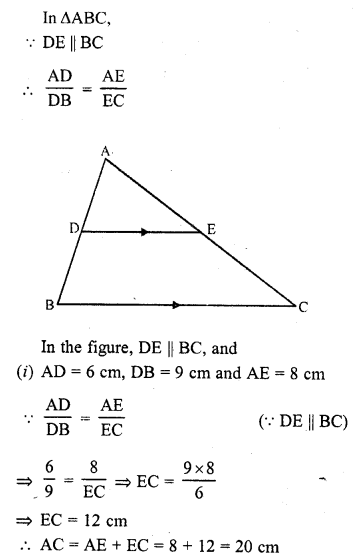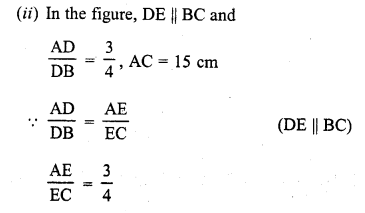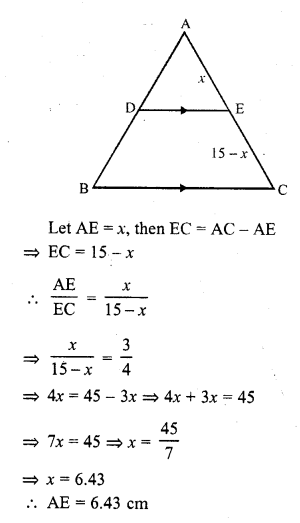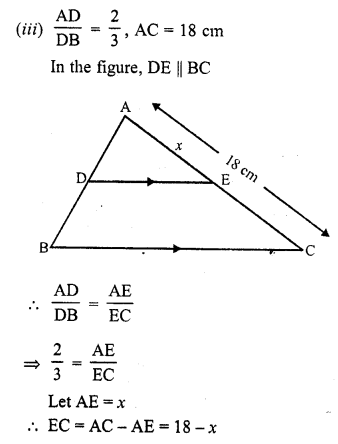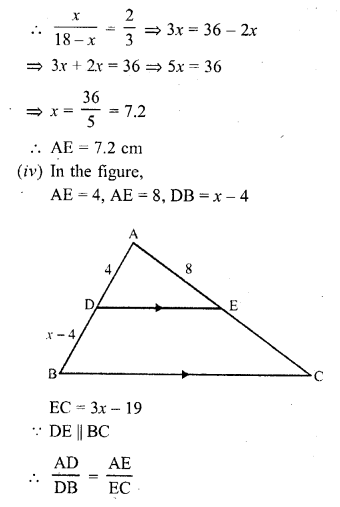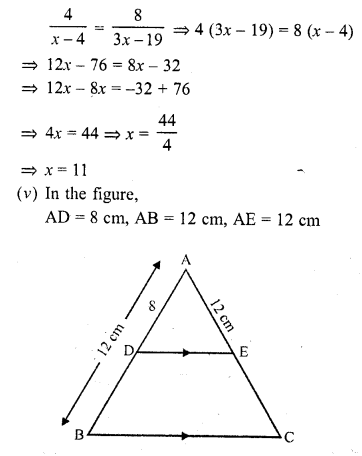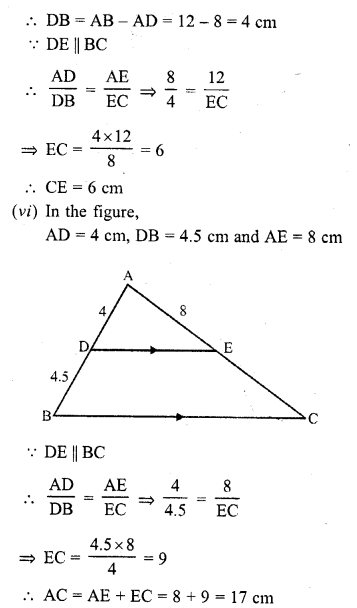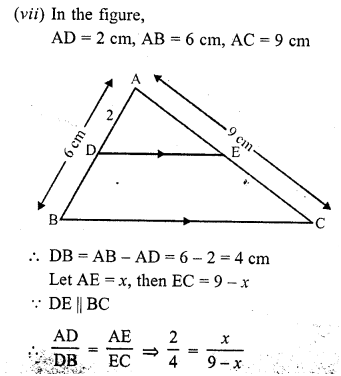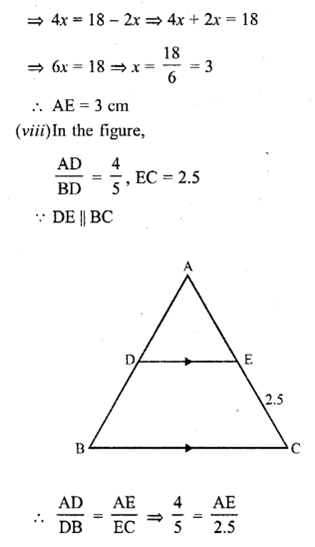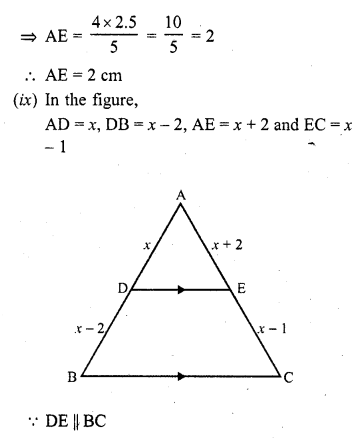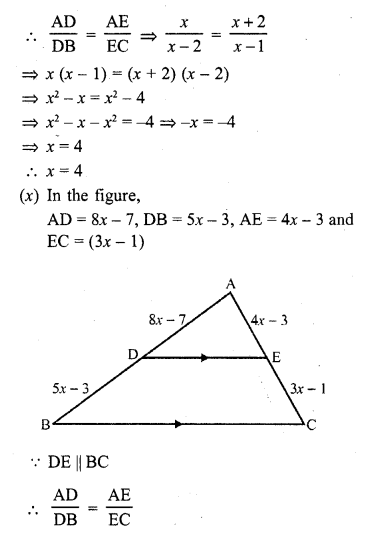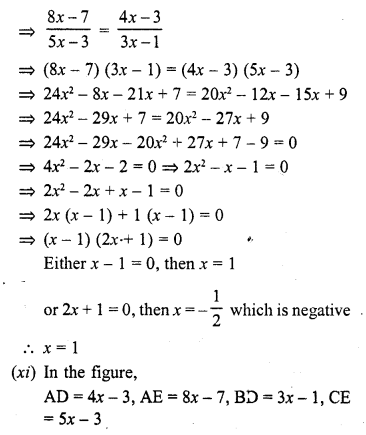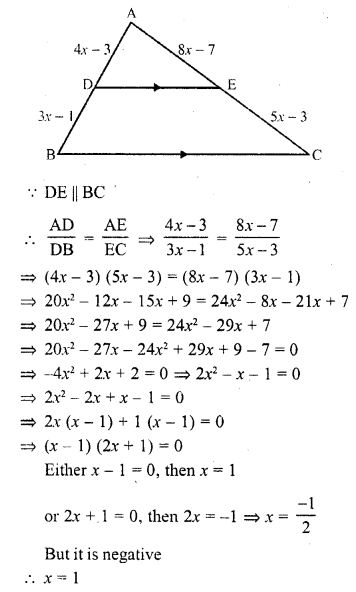• +91 9971497814
• info@interviewmaterial.com

# RD Chapter 4- Triangles Ex-4.2 Interview Questions Answers

### Related Subjects

Question 1 :
In a ∆ABC, D and E are points on the sides AB and AC respectively such that DE || BC.
(i) If AD = 6 cm, DB = 9 cm and AE = 8 cm, find AC. (C.B.S.E. 1995)
(ii) If AD/DB = 3/4 and AC = 15 cm, find AE. (C.B.S.E. 1994)
(iii) If AD/DB = 2/3 and AC = 18 cm, find AE. (C.B.S.E. 1994C)
(iv) If AD = 4, AE = 8, DB = x – 4, and EC = 3x – 19, find x. (C.B.S.E. 1992C)
(v) If AD = 8 cm, AB = 12 cm and AE = 12 cm, find CE. (C.B.S.E. 1992C)
(vi) If AD = 4 cm, DB = 4.5 cm and AE = 8 cm, find AC. (C.B.S.E. 1992C)
(vii) If AD = 1 cm, AB = 6 cm and AC = 9 cm, findAE. (C.B.S.E. 1992C)
(viii) If AD/DB = 4/5 and EC = 2.5 cm, find AE.
(ix) If AD = x, DB = x – 2, AE = x + 2 and EC = x – 1, find the value of x. (C.B.S.E. 1993C)
(x) If AD = 8x – 7, DB = 5x – 3, AE = 4x – 3 and EC = (3x – 1), find the value of x.
(xi) If AD = 4x – 3, AE = 8x – 7, BD = 3x – 1 and CE = 5x – 3, find the value of x. (C.B.S.E. 2002)
(xii) If AD = 2.5 cm, BD = 3.0 cm and AE = 3.75 cm, find the length of AC. (C.B.S.E. 2006C)